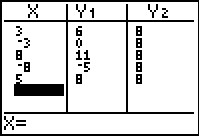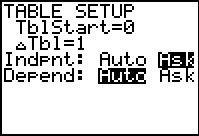••• ##### Device
• TI-83 Plus Family
• TI-84 Plus
• TI-84 Plus Silver Edition
•TI-84 Plus C Silver Edition
•TI-84 Plus CE
• ##### Report an Issue

Algebra I: One Step at a Time
by Texas Instruments#### Overview

Students solve one-step equations involving addition and multiplication by substituting possible values of a variable.

#### Key Steps

•Using the Table feature, students begin the activity by testing values for x in the equation x + 3 = 8, looking for the value of x that makes the equation true. They will practice finding the solution with several equations that contain only addition.

•By observing the solutions to many equations of the same form, students gather data to form a hypothesis about the solving an equation of this form. They will also form and solve their own equations to complete their understanding. Students are introduced to the Subtraction Property of Equality.

•At the end of the activity, students turn their attention to one-step equations involving operations other than addition. They will be introduced to the Division Property of Equality and the concept of inverse operations. Students will conclude by formulating a general rule for solving any one-step equation.